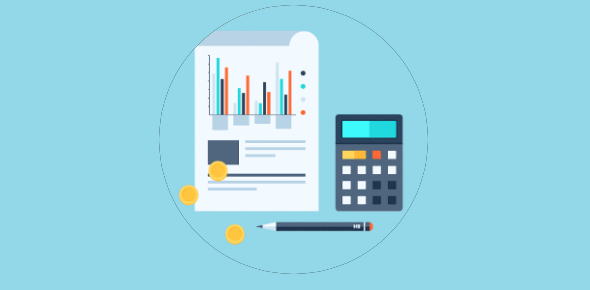# Accounting For Material Quiz: Test!

20 Questions | Total Attempts: 2342Settings.

• 1.
Direct material is a
• A.

Fixed cost

• B.

Variable cost

• C.

Semi variable cost

• 2.
Direct material is a
• A.

Manufacturing cost.

• B.

• C.

Selling and distribution cost

• D.

Any of the above

• 3.
In most industries, the most important element of cost is
• A.

Material

• B.

Labour

• C.

• 4.
Which of the following is an accounting record?
• A.

Bill of Materials

• B.

Bin card

• C.

Stores ledger

• D.

All of these

• 5.
Which of the following documents is used for issuing materials to production departments?
• A.

Purchase Requisition Note

• B.

Stores requisition Note

• C.

• D.

Stores Credit Note

• 6.
Which of the following is considered to be the normal loss of materials?
• A.

(a) Loss due to accidents

• B.

(b) Pilferage

• C.

(c) Loss due to breaking the bulk

• D.

(d) Loss due to careless handling of materials

• E.

(e) All of these

• 7.
According to which of the following methods of pricing issues are close to current economic values?
• A.

(a) Last-in-first-out price

• B.

(b) First-in-first-out price

• C.

(c) Highest-in-first-out price

• D.

(d) Weighted average price

• 8.
In which of the following methods of pricing, costs log behind the current economic values?
• A.

(a) Last-in-first-out price

• B.

(b) First-in-first-out price

• C.

(c) Replacement price

• D.

(d) Weighted average price

• 9.
Which of the following methods of stock control aims at concentrating efforts on selected items of materials?
• A.

(a) Perpetual inventory system

• B.

(b) Materials turnover

• C.

(c) Maximum, minimum and re-order level setting

• D.

(d) ABC analysis

• 10.
In base stock method of pricing the material issues, the term ‘base stock’ represents the
• A.

(a) Quantity of stock being issued

• B.

(b) Stock in balance

• C.

(c) Minimum stock

• D.

(d) Maximum stock

• 11.
Which of the following items of cost should NOT be treated as direct material ?
• A.

(a) Electricity representing 90% of the total cost.

• B.

(b) Sand paper used in production

• C.

(c) Thread used in attaching garments

• D.

(d) All of the above

• 12.
Continuous stock-taking is a part of
• A.

(a) Annual stock-taking

• B.

(b) Perpetual inventory

• C.

(c) ABC analysis.

• 13.
The classification of items in ABC analysis is made on the basis of
• A.

(a) Investment value of materials

• B.

(b) Consumption value of materi

• C.

(c) Quantity of materials consumed

• D.

(d) All of these.

• 14.
In which of the following methods, issues of materials are priced at a pre-determined rate ?
• A.

(a) Inflated price method.

• B.

(b) Standard price method

• C.

(c) Replacement price method

• D.

(d) Specific price method.

• 15.
In which of the following methods, materials are issued at the price prevailing at the time of issue ?
• A.

(a) Inflated price method

• B.

(b) Standard price method

• C.

(c) Replacement price method

• D.

(d) Specific price method.

• 16.
The storekeeper should initiate a purchase requisition when stock reaches.
• A.

(a) Minimum level

• B.

(b) Maximum level

• C.

(c) Re-order level

• D.

(d) Average level.

• 17.
Which of the following material losses should be transferred to Costing Profit and Loss Account ?
• A.

(a) Loss by evaporation

• B.

(b) Loss due to improper storage of materials

• C.

(c) Loss due to breaking the bulk

• D.

(d) All of these.

• 18.
When material prices fluctuate widely, the method of pricing that gives absurd results is
• A.

(a) Simple average price

• B.

(b) Weighted average price

• C.

(c) Moving average price

• D.

(d) Inflated price.

• 19.
When price fluctuates widely, the method that will smooth out the effect of fluctuations is
• A.

(a) Simple average

• B.

(b) Weight average

• C.

(c) FIFO

• D.

(d) LIFO

• 20.
In which of the following methods, the charge to production is not at actual cost?
• A.

(a) Weighted average price

• B.

(b) Standard price

• C.

(c) Replacement price

• D.

(d) All of these

Related TopicsBack to top